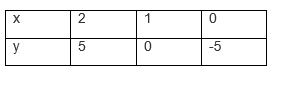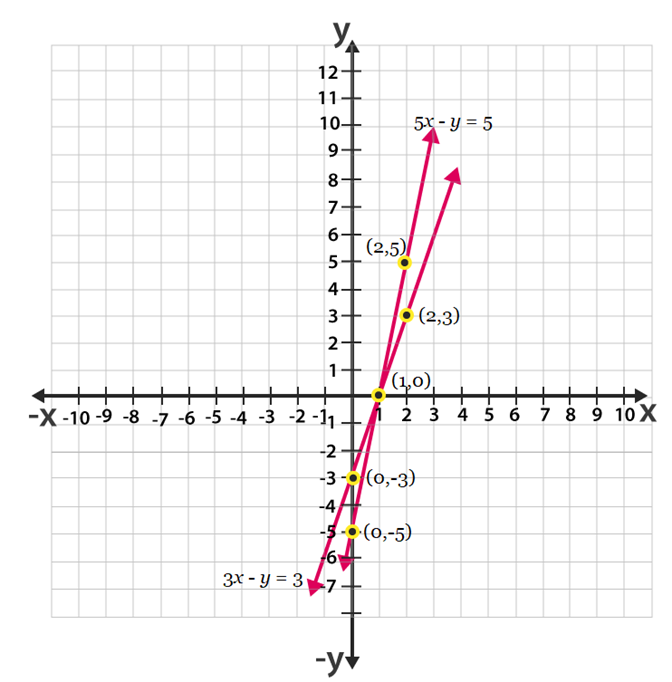Guru

# Draw the graphs of the equations 5x – y = 5 and 3x – y = 3. Determine the co-ordinates of the vertices of the triangle formed by these lines and the y axis. Q.6

• 0

The optional chapter of class 10th math of Pair of linear equations in two variables, how to solve this problem if i ever solve this question, i want good solution so please help me. Draw the graphs of the equations 5x – y = 5 and 3x – y = 3. Determine the co-ordinates of the vertices of the triangle formed by these lines and the y axis.

Share

1. Given,

5x – y = 5

=> y = 5x – 5

Its solution table will be.Also given,3x – y = 3

y = 3x – 3The graphical representation of these lines will be as follows:From the above graph we can see that the triangle formed is ∆ABC by the lines and the y axis. Also the coordinates of the vertices are A(1,0) ,  C(0,-5) and B(0,-3).

• 0